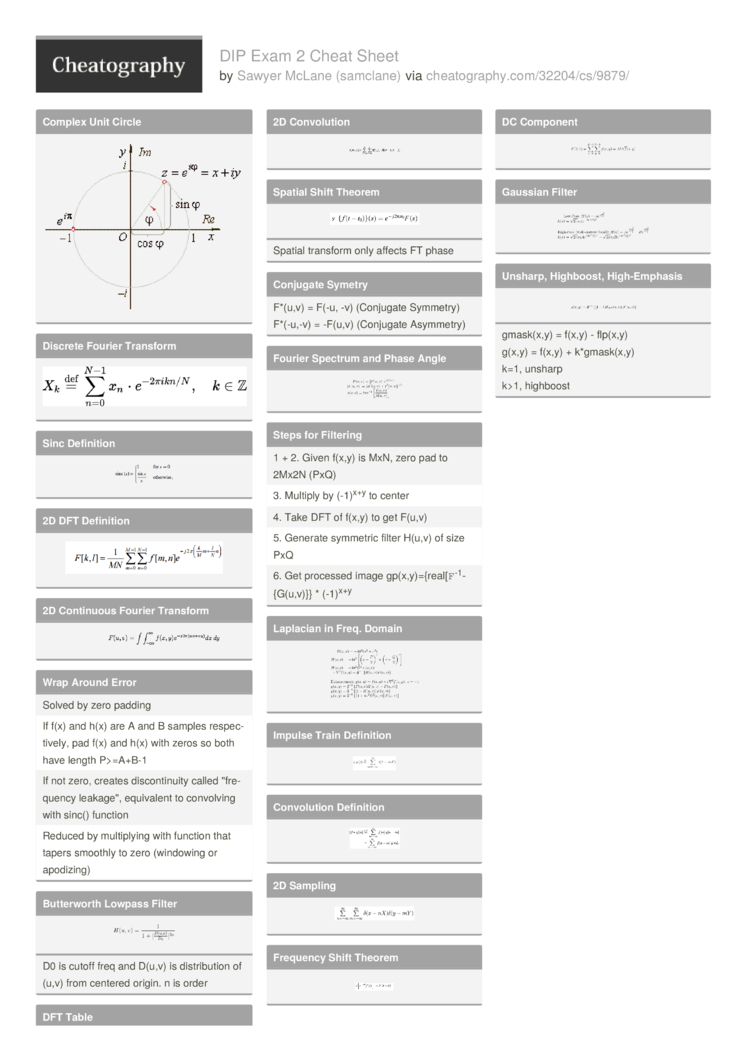# DIP Exam 2 Cheat Sheet by samclane

Digital Image Processing Exam 2 Cheat Sheet

### Complex Unit Circle### Discrete Fourier Transform### Sinc Definition### 2D DFT Definition### 2D Continuous Fourier Transform### Wrap Around Error

 Solved by zero padding If f(x) and h(x) are A and B samples respec­tively, pad f(x) and h(x) with zeros so both have length P>=­A+B-1 If not zero, creates discon­tinuity called "­fre­quency leakag­e", equivalent to convolving with sinc() function Reduced by multip­lying with function that tapers smoothly to zero (windowing or apodizing)

### Butter­worth Lowpass FilterD0 is cutoff freq and D(u,v) is distri­bution of (u,v) from centered origin. n is order

### DFT Table### Fourier Series Definition### Convol­ution TheoremSpace convol­ution = frequency multip­lic­ation

### 2D Convol­ution### Spatial Shift TheoremSpatial transform only affects FT phase

### Conjugate Symetry

 F*(u,v) = F(-u, -v) (Conjugate Symmetry) F*(-u,-v) = -F(u,v) (Conjugate Asymmetry)

### Fourier Spectrum and Phase Angle### Steps for Filtering

 1 + 2. Given f(x,y) is MxN, zero pad to 2Mx2N (PxQ) 3. Multiply by (-1)x+y to center 4. Take DFT of f(x,y) to get F(u,v) 5. Generate symmetric filter H(u,v) of size PxQ 6. Get processed image gp(x,y­)={­rea­l[`­F`­-1­{G(­u,v)}} * (-1)x+y

### Laplacian in Freq. Domain### Impulse Train Definition### Convol­ution Definition### 2D Sampling### Frequency Shift Theorem### Center DC

 To shift F(0,0) (DC Component) to center, multiply by (-1)­x+y

### Power SpectrumTotal power of image is just sum of P(u,v) over P-1,Q-1

a = 100[do­ublesum P(u,v)/Pt]

### DC Component### Gaussian Filter### Unsharp, Highboost, High-E­mphasisg(x,y) = f(x,y) + k*gmas­k(x,y)
k=1, unsharp
k>1, highboost2 Pages
//media.cheatography.com/storage/thumb/samclane_dip-exam-2.750.jpg

PDF (recommended)